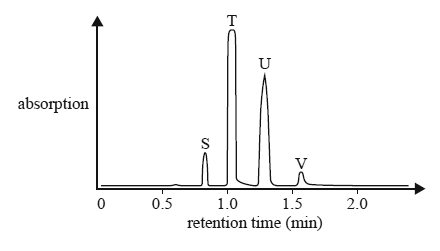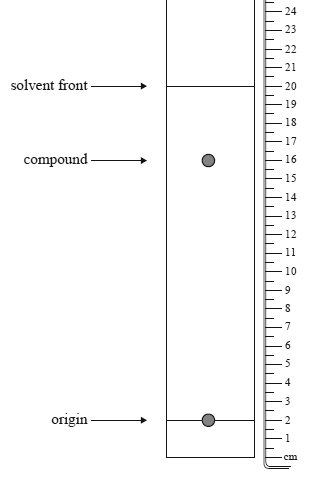Chromatography (2014 VCE) 1) Four straight chain alkanols, S, T, U, V, with a general formula ROH, were analysed using a gas chromatograph combined with a mass spectrometer. The following chromatogram was produced.What is the order of the alkanols from the highest molar mass to the lowest molar mass? A. V, U, T, S B. T, U, S, V C. V, S, U, T D. S, T, U, V Solution2) A thin layer chromatography (TLC) plate was set up with a non-polar solvent, hexane, and a polar stationary phase, silica gel. The chromatogram below was obtained. A ruler was then placed next to the plate.The Rf value for the compound would be A. 0.80 B. 0.78 C. 0.64 D. 0.61 Solution